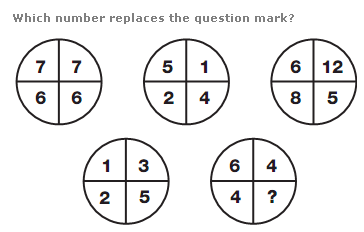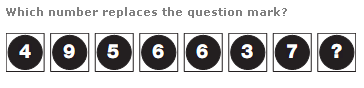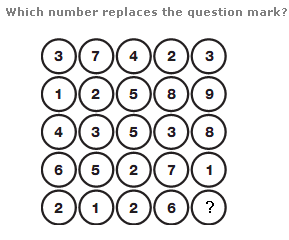# Puzzles - Number puzzles

### Exercise :: Number puzzlesAnswer : 1 Explanation : Using the numbers in the segments of the lower two circles, the numbers in the upper left circle equal the sum of the numbers in corresponding segments of the lower 2 circles, the numbers in the upper central circle equal the difference between numbers in corresponding segments of the lower 2 circles, and the numbers in the upper right circle equal the product of the numbers in corresponding segments of the lower 2 circles.Answer : 0 Explanation : Working from left to right and reading each pair of numbers as a 2 digit value, these values represent the sequence of multiples of 7, starting at 49 and finishing on 70.Answer : 3 Explanation : Work through the diagram in columns. The value in the centre of each column equals the difference between the sum of the top 2 numbers and the bottom two numbers.